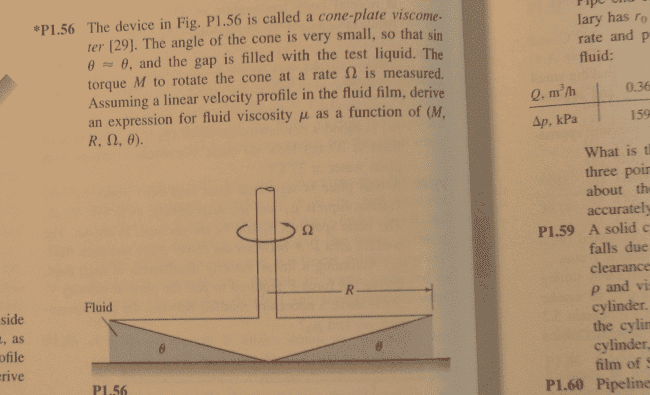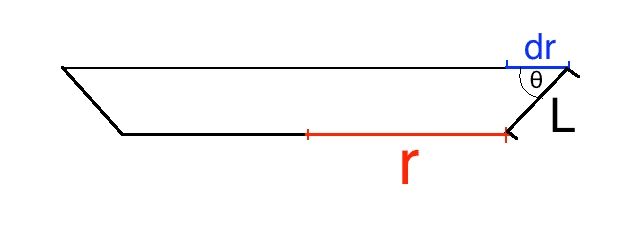# Fluid Mechanics: Cone-Plate viscometer

Feodalherren

## Homework StatementFluid Mechanics

τ =μ (du/dy)

## The Attempt at a Solution

I got far enough to write down

dM = μ (Ωr/tanθ) dA

from just substitutions, easy enough.

I get confused when I'm solving for the differential surface area. I somehow need the dA for a cone.
For a circle it's easy enough. A=πr^2 so then dA = 2πr dr.

But how do I go about getting this for a cone? the book lists it as (2πr/cosθ) dr, without any explanation, of course.

erisedk
Take a strip element 2πxdr, where x is the radius of circle formed by that strip element. Try relating x to r using similarity of triangles.

Homework Helper
Well you should first understand how to find the surface area of a "truncated cone" (ignoring the faces)
It is the average circumference of the cone multiplied by the side length (not the height)

So the surface area of a truncated cone (ignoring the faces) is $2\pi r_{avg}L$ or you could say $2\pi L\frac{r_1+r_2}{2}$
(Side note, this also applies to normal cones; just treat the tip as r2=0)

So with this understanding of truncated cones, look at the following picture I made:The differential surface area would be $2\pi r_{avg}L$ but as you can see from the picture, $L=\frac{dr}{\cos\theta}$ therefore the differential surface area is $\frac{2\pi R_{avg}}{\cos\theta}dr$

The two radii are r and (r+dr) but since dr is obviously infinitesimal, it suffices to say $r_{avg}=r$

Last edited:
•Feodalherren
erisedk
Does it really require all that? Isn't using similarity to relate x and R, considering a string element of radius x, just enough?

Homework Helper
Does it really require all that? Isn't using similarity to relate x and R, considering a string element of radius x, just enough?
No that is incorrect. The differential area element is $\frac{2\pi x}{\cos\theta}dx$

Me and the OP were using r not to represent the radius of the base of the cone, but to represent what you called "x"

erisedk
No that is incorrect. The differential area element is $\frac{2\pi x}{\cos\theta}dx$

Me and the OP were using r not to represent the radius of the base of the cone, but to represent what you called "x"

Oh ok.

Feodalherren
Well you should first understand how to find the surface area of a "truncated cone" (ignoring the faces)
It is the average circumference of the cone multiplied by the side length (not the height)

So the surface area of a truncated cone (ignoring the faces) is $2\pi r_{avg}L$ or you could say $2\pi L\frac{r_1+r_2}{2}$
(Side note, this also applies to normal cones; just treat the tip as r2=0)

So with this understanding of truncated cones, look at the following picture I made:
View attachment 78118
The differential surface area would be $2\pi r_{avg}L$ but as you can see from the picture, $L=\frac{dr}{\cos\theta}$ therefore the differential surface area is $\frac{2\pi R_{avg}}{\cos\theta}dr$

The two radii are r and (r+dr) but since dr is obviously infinitesimal, it is suffices to say $r_{avg}=r$
Excellent explanation. Thank you very much, Sir.